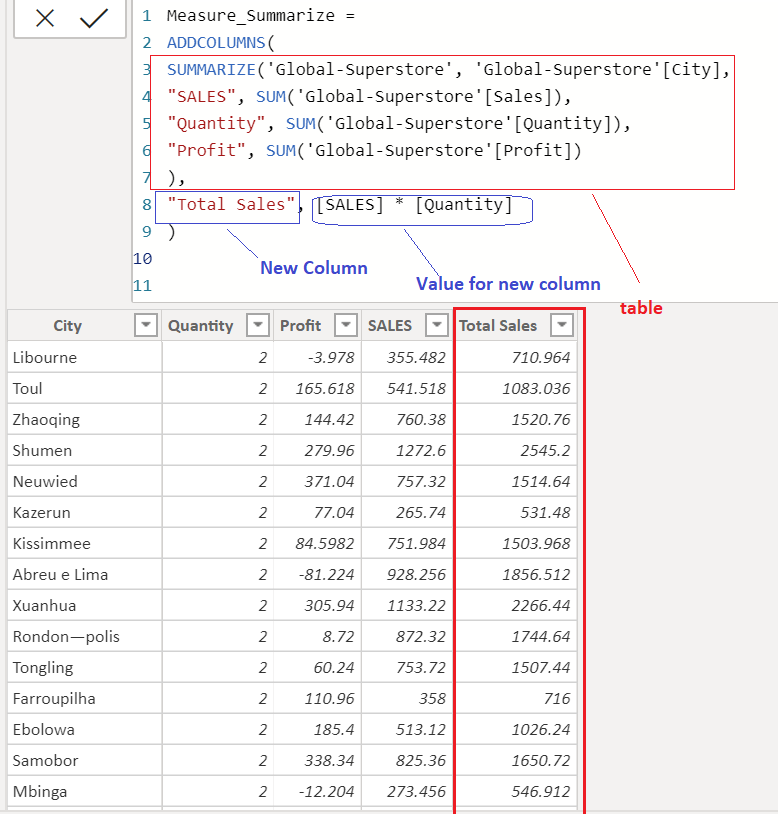### ADDCOLUMNS is a POWER BI Table Manipulation Functions in DAX which is used to add a calulated columns in table.

Basically, ADDCOUMNS returns a table with new column specified in DAX expression.

```SYNTAX
[, <column_name>, <expression>]…)```

table_name is a table or any DAX express that returns a table.
column_name is name that is specified to the column, must enclosed in double quotes.
expression is a DAX expression that returns a scalar value, It is evaluated for each rows of table.

Lets look at an example of ADDCOULMNS.

Here we have a table salesData which is created using a DATATABLE function DAX as shown below.

```SalesData =
DATATABLE(
"SalesId", INTEGER,
"Year", STRING,
"Amount_Per_Qty",CURRENCY,
"Quantity", INTEGER,
{
{1, 2017, 5000, 4600},
{2, 2018, 5500, 4590},
{3, 2018, 6000, 2345},
{4, 2020, 5600, 1000}
}
)```Now we will add a new column named “Total Sales  Amount” into this table using a ADDCOLUMNS Function. Value for “Total Sales  Amount” will be a multiplication of Amount_Per_Qty with Quantity.

Lets add a new columns into table SalesData.

```SalesData =
DATATABLE(
"SalesId", INTEGER,
"Year", STRING,
"Amount_Per_Qty",CURRENCY,
"Quantity", INTEGER,
{
{1, 2017, 5000, 4600},
{2, 2018, 5500, 4590},
{3, 2018, 6000, 2345},
{4, 2020, 5600, 1000}
}
),
"TOTAL SALES AMOUNT", [Amount_Per_Qty] * [Quantity]
)```As you can see, the new column “TOTAL SALES AMOUNT” is added to the table SalesData.

You can also use ADDCOLUMNS to add new column in Summarize table as shown below.

```Measure_Summarize =
SUMMARIZE('Global-Superstore', 'Global-Superstore'[City],
"SALES", SUM('Global-Superstore'[Sales]),
"Quantity", SUM('Global-Superstore'[Quantity]),
"Profit", SUM('Global-Superstore'[Profit])

)```Lets add new column named “Total Sales”, and value for that column will be a Quantity * SALES.

Following DAX expression uses ADDCOLUMNS to add a column into Summarize table and returns new table.

```Measure_Summarize =
SUMMARIZE('Global-Superstore', 'Global-Superstore'[City],
"SALES", SUM('Global-Superstore'[Sales]),
"Quantity", SUM('Global-Superstore'[Quantity]),
"Profit", SUM('Global-Superstore'[Profit])
),
"Total Sales", [SALES] * [Quantity]
)```Table Manipulation Functions

SUMMARIZE

SUMMARIZECOLUMNS

VALUES

DATATABLE

This site uses Akismet to reduce spam. Learn how your comment data is processed.